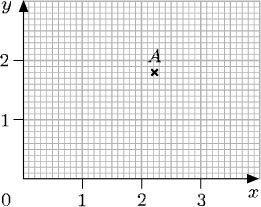A fraction is written as one number over another (such as) and means the top number divided by the bottom number. The top number, 3, is called the numerator and the bottom number, 10, is call
Author(s): The Open University

## Activity 6

What is 370.76 grams in kilograms? There are 1000 grams in a kilogram.

370.76 ÷ 1000 = 0.370 76.

So 370.76 g = 0.370
Author(s): The Open University

## Activity 2

Write each of the following three numbers in numerals and then place them in ascending order:

• eight hundred and eight thousand

• two million and twenty
Author(s): The Open University

## Activity 1

Can you answer the following questions:

• (a) Write ‘twenty thousand one hundred and forty-four’ as a number.

• (b) Say (or write) the number 31 002
Author(s): The Open University

After studying this course, you should be able to:

• write whole numbers and decimals in place-value columns and compare their sizes

• multiply and divide whole numbers and decimals by 10, 100, 1000 and so on

• indicate given fractions on a diagram and find equivalent fractions for a given fraction

• mark numbers on a number line

• choose appropriate units for a given purpose.

Author(s): The Open University

The content acknowledged below is Proprietary (see terms and conditions) and is made available under a Creative Commons Attribution-NonCommercial-ShareAlike 4.0 Licence

Grateful acknowledgement is made to the following sources for permission to reproduce material in this course:<
Author(s): The Open University## Study another free course

There are more than 800 courses on OpenLearn for you to
Author(s): The Open University

Now try the quiz and see if there are any areas you need to work on.

Author(s): The Open University

## Activity 22

Write down the coordinates of the point P on each of the graphs below and interpret these coordinates in terms of the labels on the axes.

Author(s): The Open University

## Activity 20

Look at the diagram below and answer the following questions:

• (a) Write down the coordinates of the points P, Q, R, S and T.

Author(s): The Open University

Where necessary, the coordinates of a point can be specified by using decimals or fractions. However, locating points whose coordinates are not whole numbers requires more precision when reading along the axes. For example, here the coordinates of the point A are (2.2, 1.8).Author(s): The Open University

Up to now only those points with positive or zero coordinates have been considered. But the system can be made to cope with points involving negative coordinates, such as (−2, 3) or (−2, −3). Just as a number line can be extended to deal with negative numbers, the x-axis and y-axis can be extended to deal with negative coordinates.

[Image_Link]https://www.open.edu/openlearn/ocw/pluginfile.php/94235/mod_oucont
Author(s): The Open University

## Activity 16

Write down the coordinates of A and B.

[Image_Link]https://www.open.edu/openlearn/ocw/pluginfile.php/94235/mod_oucontent/oucont
Author(s): The Open University

For many towns and cities, an individual book of street maps called an A to Z has been produced. You can look up the name of a street in the index, and it will give you the page number of the map that contains the street, plus the grid reference square for the street. There are different conventions for these grid references. You may have met several of these.

## Activity 10

This table categorises Tom's activities for the day.

Activity<
Author(s): The Open University

Pie charts are representations that make it easy to compare proportions: in particular, they allow quick identification of very large proportions and very small proportions. They are generally based on large sets of data.

The pie chart below summarises the average weekly expenditure by a sample of families on food and drink. The whole circle represents 100% of the expenditure. The circle is then divided into ‘segments’, and the area of each segment represents a fraction or pe
Author(s): The Open University

## Activity 7

Tables often give information in percentages. The table below indicates how the size of households in Great Britain changed over a period of nearly 30 years.

Number of people in household1961 (%)1971 (%)1981 (%)1991 (%)
1Author(s): The Open University

## Activity 6

The table below indicates the cooling rate of tea in a teapot.

Time/mins
Author(s): The Open University

Experiments or surveys usually generate a lot of information from which it is possible to draw conclusions. Such information is called data. Data are often presented in newspapers or books.

One convenient way to present data is in a table. For instance, the nutrition panel on the back of a food packet:

### Nutrition Information

Author(s): The Open University